# Vectors and Scalars

In Physics we will encounter two very common types of quantities: Vectors and scalars.

## Scalars

1. Definition of Scalar

Scalars are quantities which only have magnitude. Scalars can be added and subtracted like normal numbers.

1. Examples:

Quantities like mass, temperature, volume; charge, time, etc are all scalar quantities. Their magnitudes are enough to define them.

*time is not a vector quantity. It cannot go in different directions like left, right, up, down, etc.

## Vectors

• Definition of vector

Vectors are quantities that have magnitude and directions. Both these qualities together define a vector.

Examples of vector quantities are: displacement, velocity, acceleration, force, electric field, magnetic field, etc.

1. vector quantities are generally denoted with an overhead arrow (force=) or in bold letters (force=F)

Graphically vectors are represented by arrows. The relative length of arrows typically signifies the magnitude of the vector quantity while the direction towards which the arrow is pointing gives the direction of the vector.

We must remember that distance is a scalar quantity while displacement is a vector quantity.

How so? There is an example at the end of this article to clarify this notion.

## Graphical representation

1. Representation by arrows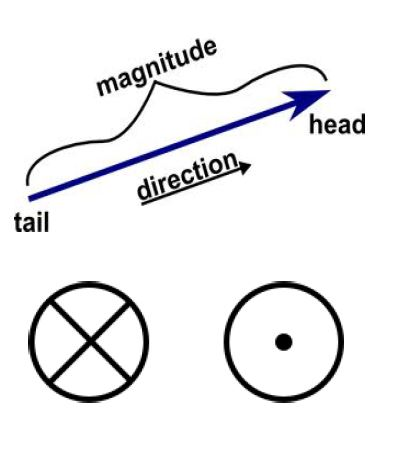As mentioned earlier the length of the arrow represents the strength of the vector and the direction at which the arrow-head points, gives the direction of the vector.

Vectors pointing into the plane of paper are typically represented by a cross and vectors pointing out of the plane of paper are typically represented by a point.

1. Now suppose we have two vectors represented by arrows a (pointing north) and B (pointing west). If we shift these arrows a little without changing their direction or length, we see, in their new position the arrows still point towards the same direction (A-north and B-west) and have the same length. Thus the vectors represented by them also have the same direction and magnitude.

So we see that we can move around vectors as long as we don’t change their length (magnitude) or direction.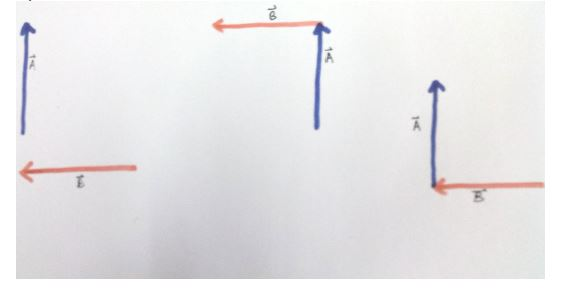If we need to add vector V1 and vector V2 we just need to line up the tail of vector V2 to the head of vector V1. Since we are allowed to move or shift vectors (as long as we don’t change the length or direction of the vector), this is a very simple task. Then if we draw a resultant arrow starting from the tail of V1 and ending at the head of V2, this resultant vector arrow (in purple colour) represents the vector sum of V1+V2.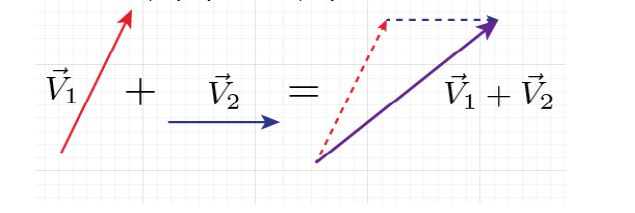• Law of parallelogram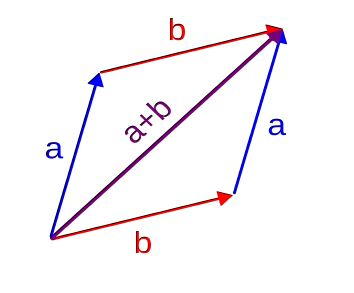The law of parallelogram beautifully demonstrates vector addition.

Now, say, as in the figure we have two vectors, a (blue arrow) and b (red arrow). We can add them as we discussed above by lining up the tail of b to the head of a and the resultant vector a + b is the vector denoted by the purple arrow.

Now, what if we go the other way round and place the tail of a to the head of b?

We will end up with the same resultant vector!

This beautifully demonstrates also that a+b=b+a , i.e. vector addition is commutative.

We also see that the diagram sketched in the process with vector a and b is parallelogram. So if we have to add a and b, we can simply draw them starting from the same point (say point ‘O’) and with these two vectors as two adjacent sides if we draw a complete parallelogram, then the diagonal from the point ‘O’ to the opposite corner is the resultant vector. This is the law of parallelogram.

## Graphical subtraction

1. Negative of a vector

Negative of a vector is simply the vector of the same magnitude in the opposite direction. Thus graphically we just need to rotate the arrow by 180 degrees.

So now if need to subtract b from a instead of writing it as ab we write it as a+(-b) and add negative of vector b to a.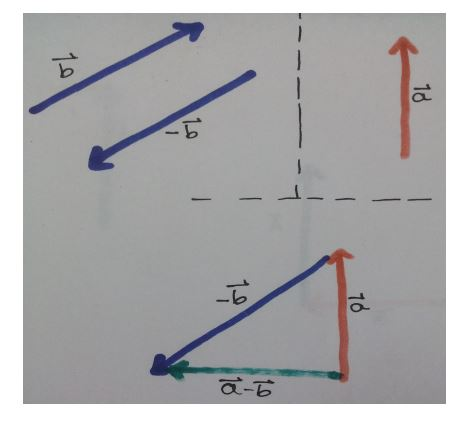So, ab = a+(-b) = c

## Vector components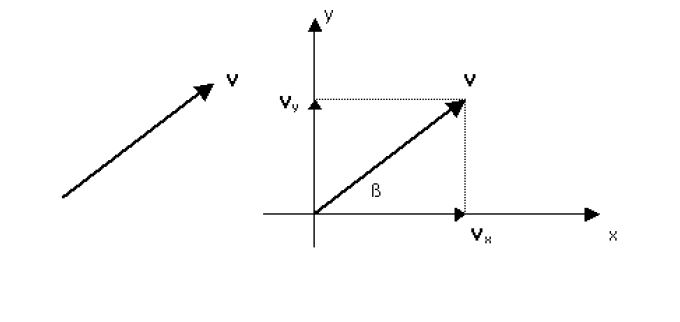1. Let’s say we have vector In a x-y co-ordinate plane, we can express V as the sum of Vx (a vector along x axis) and Vy (a vector along y axis).

When we do so, the vectors Vx and Vy are known as components of V along x axis and y axis respectively.

This makes operation involving vectors quite easy.

How do we figure out the components?

As we see the x component of the vector is along the x axis and the y component is along the y axis. So we already have the direction. Now we see that the vectors V, Vx and Vy make a right angles triangle and the angle between V and Vx is ?.

Using basic trigonometry we see that Vx/V = cos ?

So, Vx = V cos ?

Similarly Vy = V sin ?

If we know the components of vectors, we can simply add the components and find out the resultant vectors instead of drawing the two vectors and figuring it out.

EX: say we have vector A with components Ax and Ay long x and y axis. Vector B with components Bx and By along x and y axis. We notice that the components of resultant vector have a x-axis component equal to Ax+Bx and y-axis component equal to Ay+By.

So if the resultant vector is R with components Rx and Ry.

Then Rx=Ax+Bx

and Ry = Ay+By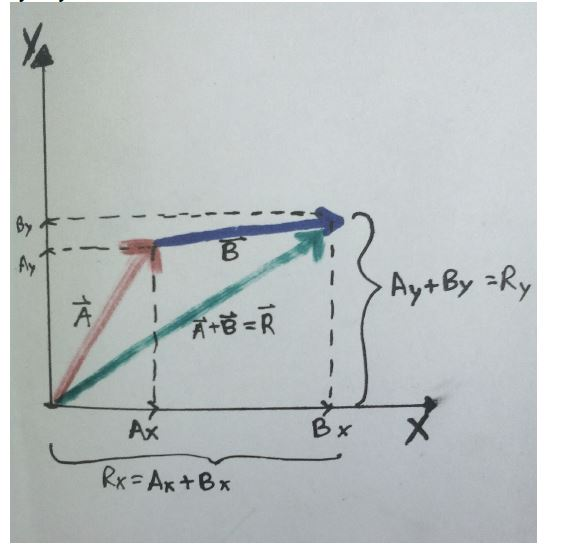## Angle of a vector

Now if we are given the components of a vector, figuring out the angle from inverse trigonometric functions is very easy.

We know that Vx = V cos ?

So ? = cos-1(Vx/V)

Similarly ? = sin-1(Vy/V)

and ? = tan-1(Vy/Vx)

## Vector notation

1. Vectors can be expressed in many ways. We have already seen how to express them graphically using arrows in 2 dimension.

We also say how it can be expressed as sum of components of x and y axis.

• We can extend this to 3D. Then the vectors will have components along x, y and z axis

This can be expressed in a mathematical notation as i, j, k . V = <Vx, Vy, Vz>. Where Vx, Vy and Vz are the x, y and z axis components respectively.

• Or we can introduce unit vectors called i, j, k , which are unit vectors along x, y and z axis.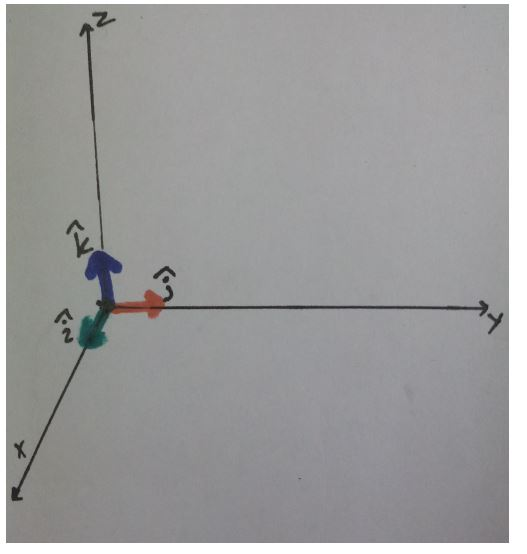Then vectors can be expressed as V = Vxi + Vyj + Vzk

• Then as explained earlier for 2dimension vectors, we can simply add vectors by adding their respective components.

So if we want to add two vectors P and Q, where P=<2, 4, 6> and Q=<4, 5, 6>. We simply add their components. P+Q = <2+4, 4+5, 6+6> = <6, 9, 12>.

So the resultant vector is R=<6, 9, 12>

## Now let’s see some examples:

1. Let’s say a woman walks 3m to the east and 4m to the north. What is the distance covered and what is her displacement?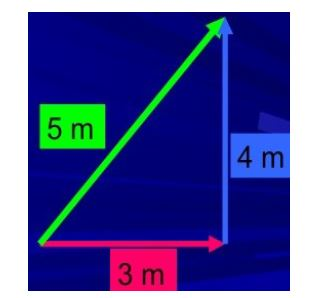Answer: As we can see the distance travelled is 3m+4m = 7m. Distance is a scalar quantity and gets added like normal numbers.

The displacement on the other hand is vector. It is the net movement between starting point and the ending point. The green arrow represents the net displacement as vector.

If we apply pythagoras theorem then the length of the green arrow is

Thus displacement is 5m towards the north-eastern side.

[box type=”success” align=”” class=”” width=””]For more examples check out some of the lessons in our AP Physics 1 & 2 course. We cover topics such as Vectors & Scalars.[/box]

SPECIAL OFFER!

`SUCCESS8`